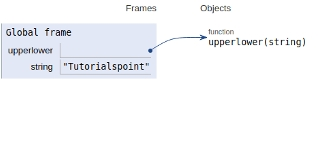# Python program to count upper and lower case characters without using inbuilt functions

In this article, we will learn about the solution and approach to solve the given problem statement.

## Problem statement

Given a string input, we need to find the number of uppercase & lowercase characters in the given strings.

Here we will we checking ASCII value of each character by the help of built-in ord() function.

Here we have assigned two counters to 0 and we are traversing the input string and checking their ASCII values and incrementing their counter respectively.

Now let’s see the implementation below −

## Example

Live Demo

def upperlower(string):
upper = 0
lower = 0
for i in range(len(string)):
# For lowercase
if (ord(string[i]) >= 97 and
ord(string[i]) <= 122):
lower += 1
# For uppercase
elif (ord(string[i]) >= 65 and
ord(string[i]) <= 90):
upper += 1
print('Lower case characters = %s' %lower,
'Upper case characters = %s' %upper)
# Driver Code
string = 'Tutorialspoint'
upperlower(string)

## Output

Lower case characters = 13 Upper case characters = 1

All variables and functions are declared in global scope as shown in the figure below.## Conclusion

In this article, we learned about the approach to count upper and lower case characters without using inbuilt functions.